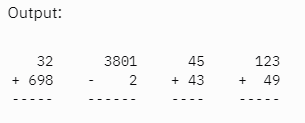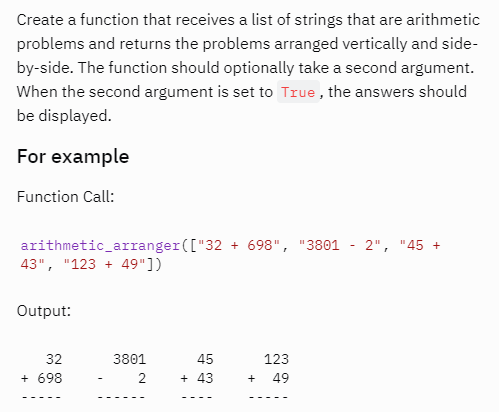# Python arithmetic formatter - string format issues

I am trying to format each calculation using new list i.e regex_split. format seems to work when I use i substitute 1 in for 0 so I am not sure why it doesn’t work for the first list item.

`````` if len(regex_split) > len(regex_split[-1]):

regex_split = f'{regex_split:>2}'
regex_split = f'{regex_split:>{len(regex_split)}}
``````

Could you say something more? What do you expect to happen, what is happening instead? Some examples when it works and not?

Trying to add spaces next to the numbers in the array:

[’ 32’, ‘-’, ’ 698’] - do all of that to get final result looking similar to these calculations belowBut what is `regex_split`? How is it used later? You have mentioned it works sometimes, for what cases it does? For what it doesn’t? Without more context what pasted code should be doing, and how that fits into the rest of code, it may be hard if not impossible to help.

regit _split is a list made up of the pieces that make up a calculation. eg 32 + 698 becomes regex_split = [‘32’, ‘+’, ‘698’]. I then try to edit each item to add the spaces required to look like the image above.``````nstr = ["32 + 698", "3801 - 2", "45 + 43", "123 + 49"]
for i in nstr:
ns = i.split()
n1 = int(ns)
n2 = int(ns)
if ns == '+':
print(ns,ns,ns,'=',n1+n2)
if ns == '-':
print(ns,ns,ns,'=',n1-n2)
``````

You can easily achieve this using the default list. As we know there are going to be only 3 items under each list item and we know the first and the third are numbers, you can just use the index to perform the operation:

``````>>> nstr.split()
['32', '+', '698']
``````

This topic was automatically closed 182 days after the last reply. New replies are no longer allowed.